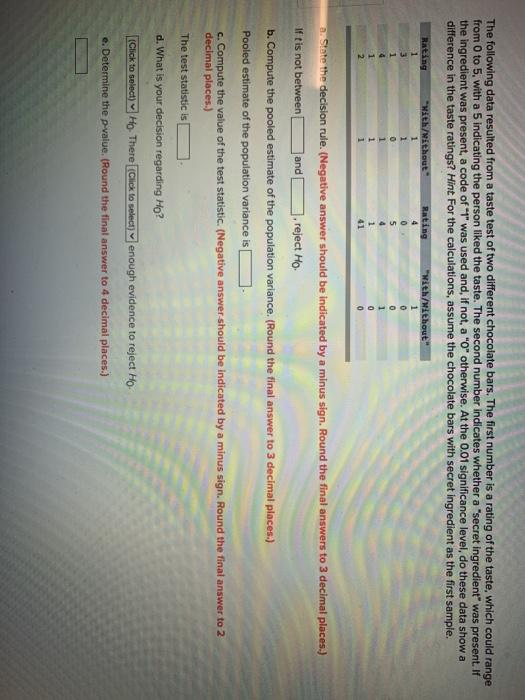# Question Solved1 AnswerThe following data resulted from a taste test of two different chocolate bars. The first number is a rating of the taste, which could range from 0 to 5, with a 5 indicating the person liked the taste. The second number indicates whether a "secret ingredient was present. If the ingredient was present, a code of "1" was used and, if not, a "0" otherwise. At the 0.01 significance level, do these data show a difference in the taste ratings? Hint For the calculations, assume the chocolate bars with secret ingredient as the first sample. Rating "With/without Rating 1 3 "With/without 1 1 0 0 5 4 1 41 a.state the decision rule. (Negative answer should be indicated by a minus sign. Round the final answers to 3 decimal places.) Iftis not between and reject Ho b. Compute the pooled estimate of the population variance. (Round the final answer to 3 decimal places.) Pooled estimate of the population variance is c. Compute the value of the test statistic. (Negative answer should be indicated by a minus sign. Round the final answer to 2 decimal places.) The test statistic is d. What is your decision regarding Ho? (Click to select) Ho. There (Click to select)y enough evidence to reject Ho e. Determine the p value. (Round the final answer to 4 decimal places.)4SID3H The Asker · Probability and StatisticsTranscribed Image Text: The following data resulted from a taste test of two different chocolate bars. The first number is a rating of the taste, which could range from 0 to 5, with a 5 indicating the person liked the taste. The second number indicates whether a "secret ingredient was present. If the ingredient was present, a code of "1" was used and, if not, a "0" otherwise. At the 0.01 significance level, do these data show a difference in the taste ratings? Hint For the calculations, assume the chocolate bars with secret ingredient as the first sample. Rating "With/without Rating 1 3 "With/without 1 1 0 0 5 4 1 41 a.state the decision rule. (Negative answer should be indicated by a minus sign. Round the final answers to 3 decimal places.) Iftis not between and reject Ho b. Compute the pooled estimate of the population variance. (Round the final answer to 3 decimal places.) Pooled estimate of the population variance is c. Compute the value of the test statistic. (Negative answer should be indicated by a minus sign. Round the final answer to 2 decimal places.) The test statistic is d. What is your decision regarding Ho? (Click to select) Ho. There (Click to select)y enough evidence to reject Ho e. Determine the p value. (Round the final answer to 4 decimal places.)
More
Transcribed Image Text: The following data resulted from a taste test of two different chocolate bars. The first number is a rating of the taste, which could range from 0 to 5, with a 5 indicating the person liked the taste. The second number indicates whether a "secret ingredient was present. If the ingredient was present, a code of "1" was used and, if not, a "0" otherwise. At the 0.01 significance level, do these data show a difference in the taste ratings? Hint For the calculations, assume the chocolate bars with secret ingredient as the first sample. Rating "With/without Rating 1 3 "With/without 1 1 0 0 5 4 1 41 a.state the decision rule. (Negative answer should be indicated by a minus sign. Round the final answers to 3 decimal places.) Iftis not between and reject Ho b. Compute the pooled estimate of the population variance. (Round the final answer to 3 decimal places.) Pooled estimate of the population variance is c. Compute the value of the test statistic. (Negative answer should be indicated by a minus sign. Round the final answer to 2 decimal places.) The test statistic is d. What is your decision regarding Ho? (Click to select) Ho. There (Click to select)y enough evidence to reject Ho e. Determine the p value. (Round the final answer to 4 decimal places.)